# Cost price of Products

## How are cost prices used in Sumtracker

Cost prices are used in reports for cost of goods sold and stock valuation. When you export product list or stock by location, the cost per unit and total cost value is exported for each product.

Cost Prices can be updated through the following ways

• manually updated in products
• automatic update through Purchase Orders

## Update cost price of products in bulk

Step 1 - Export the Product List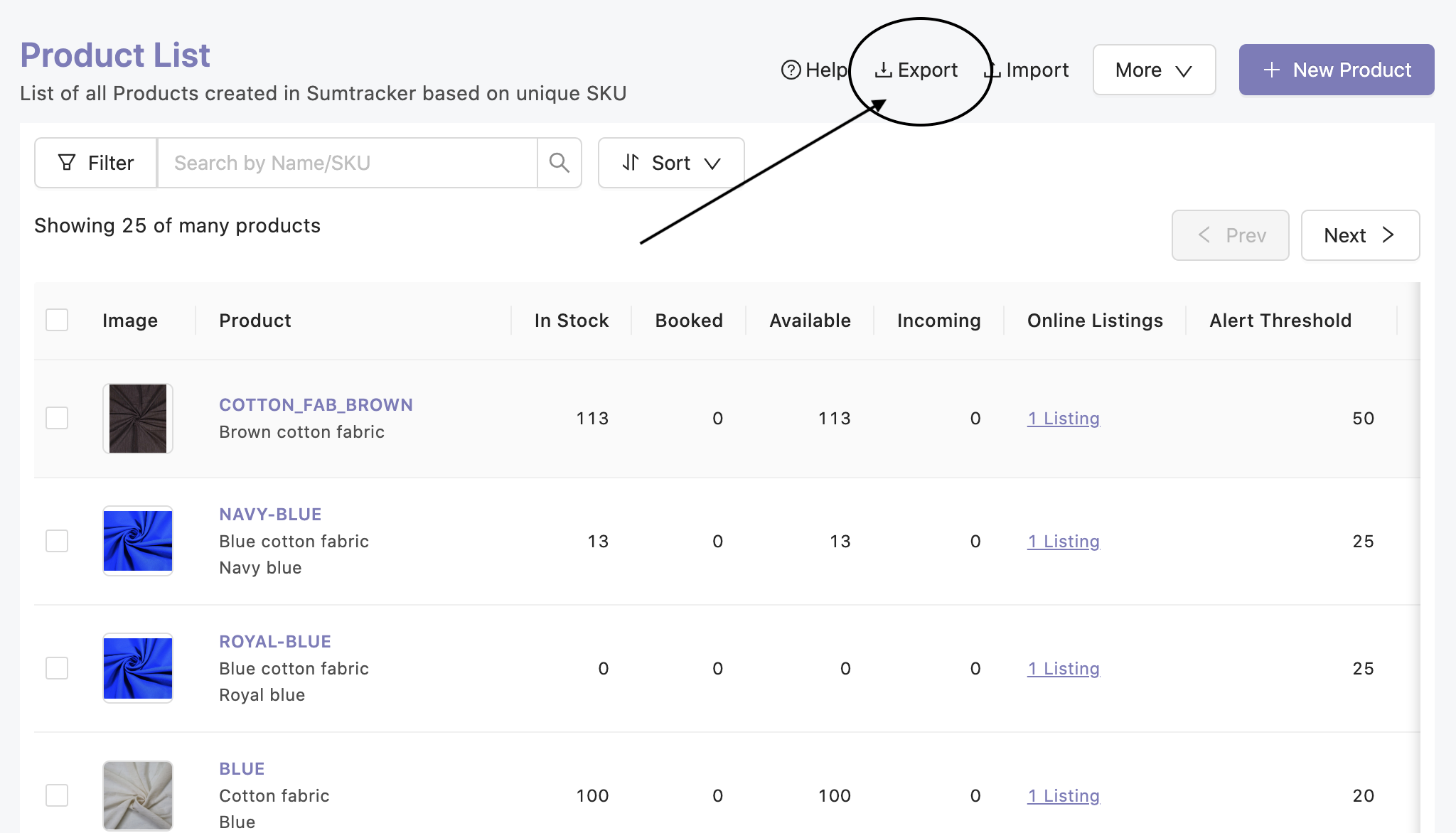Step 2 - Add Cost corresponding the Product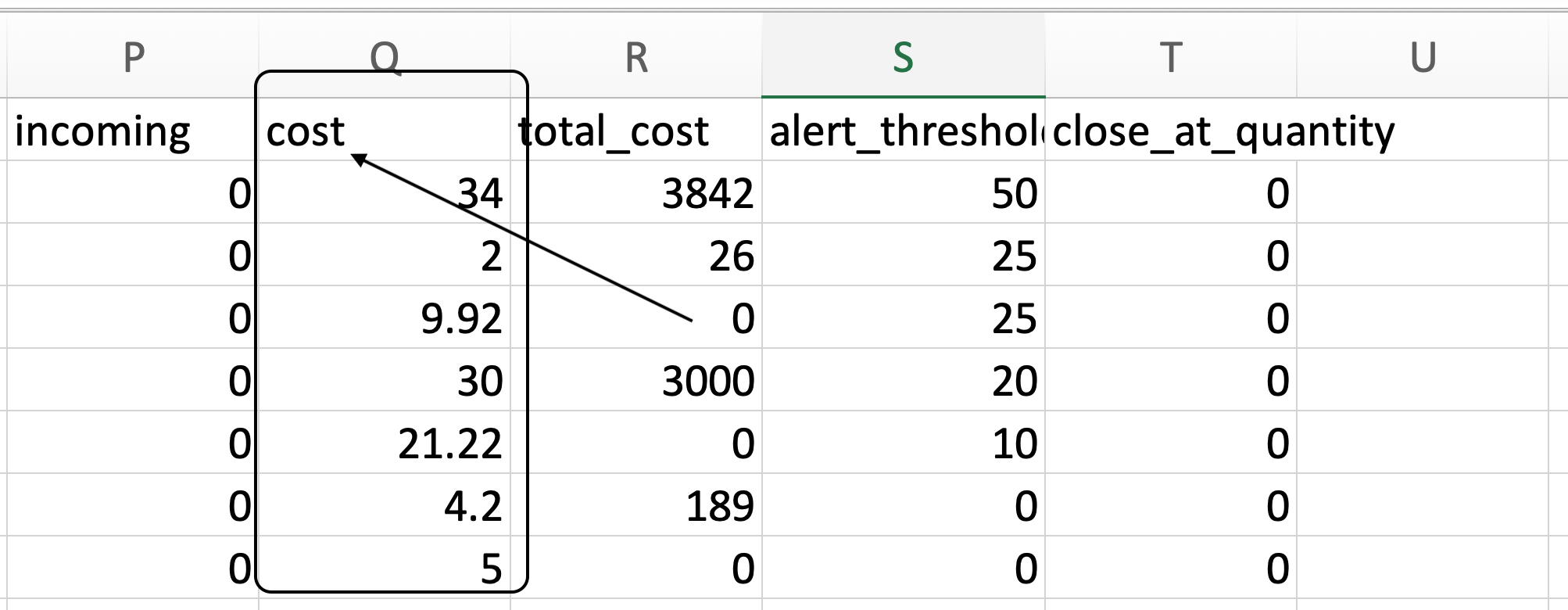Step 3 - Import the List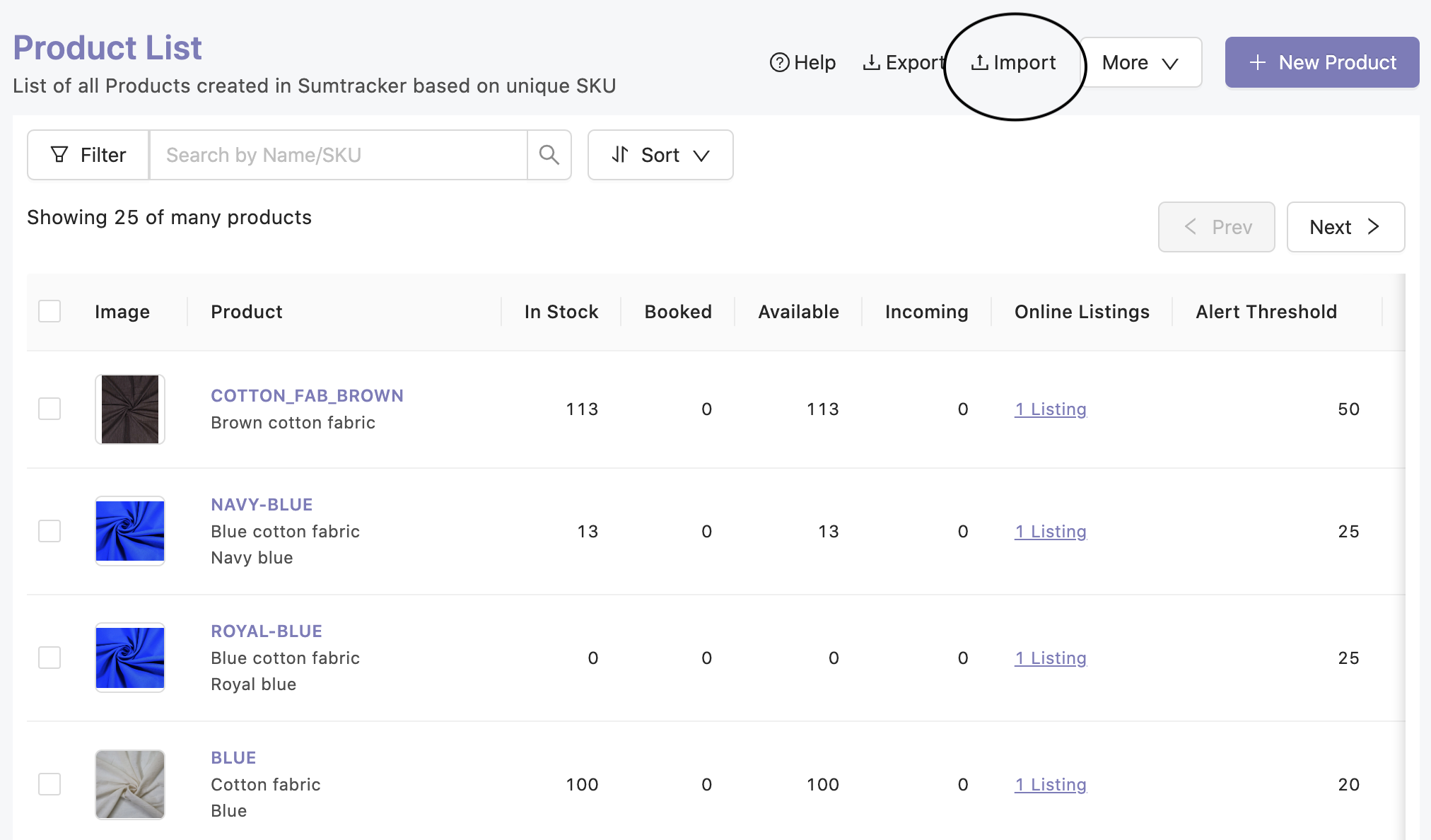## Update cost price of products individually

Step 1: Click on Product SKU in Product List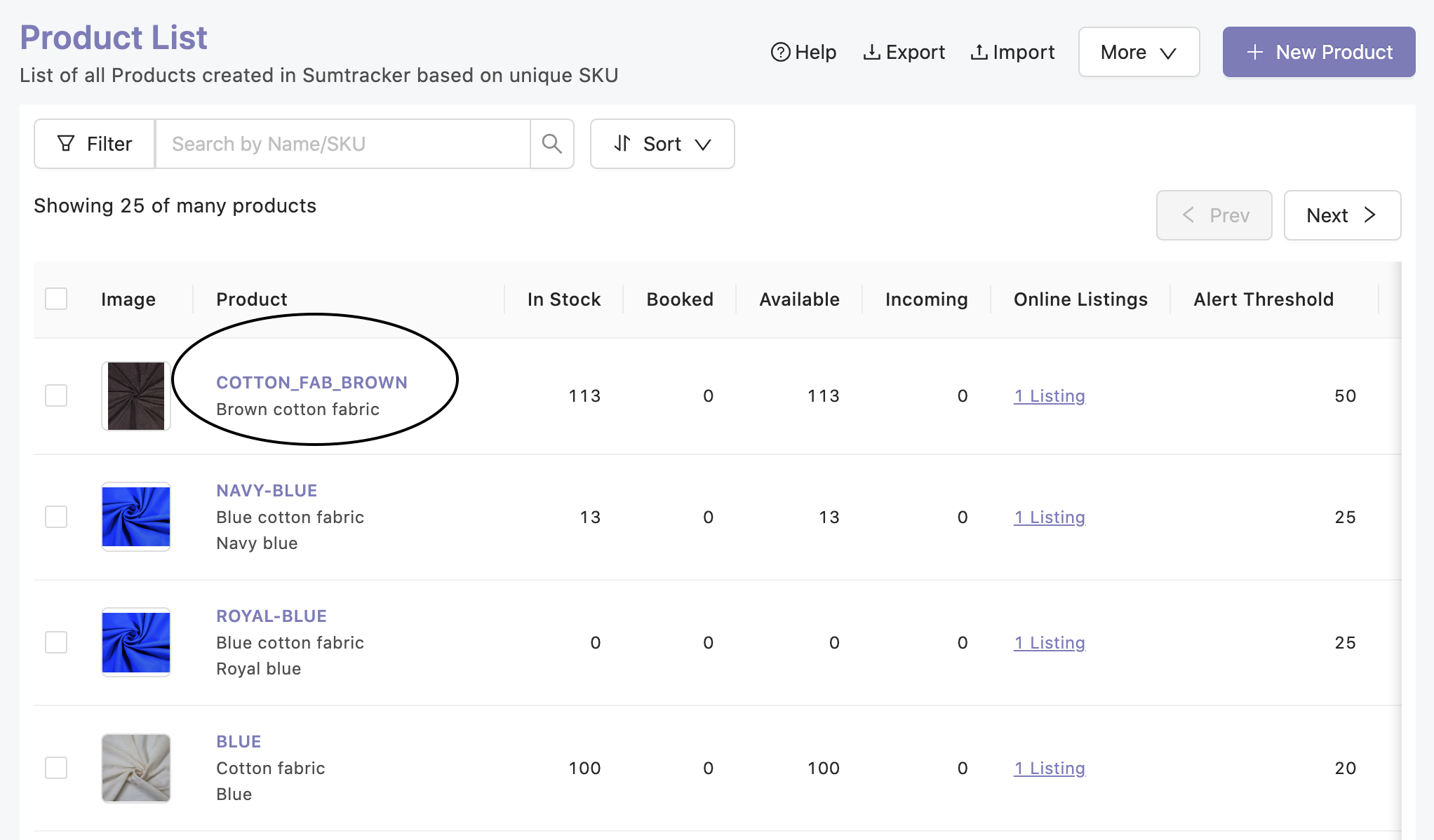Step 2: add cost and save the product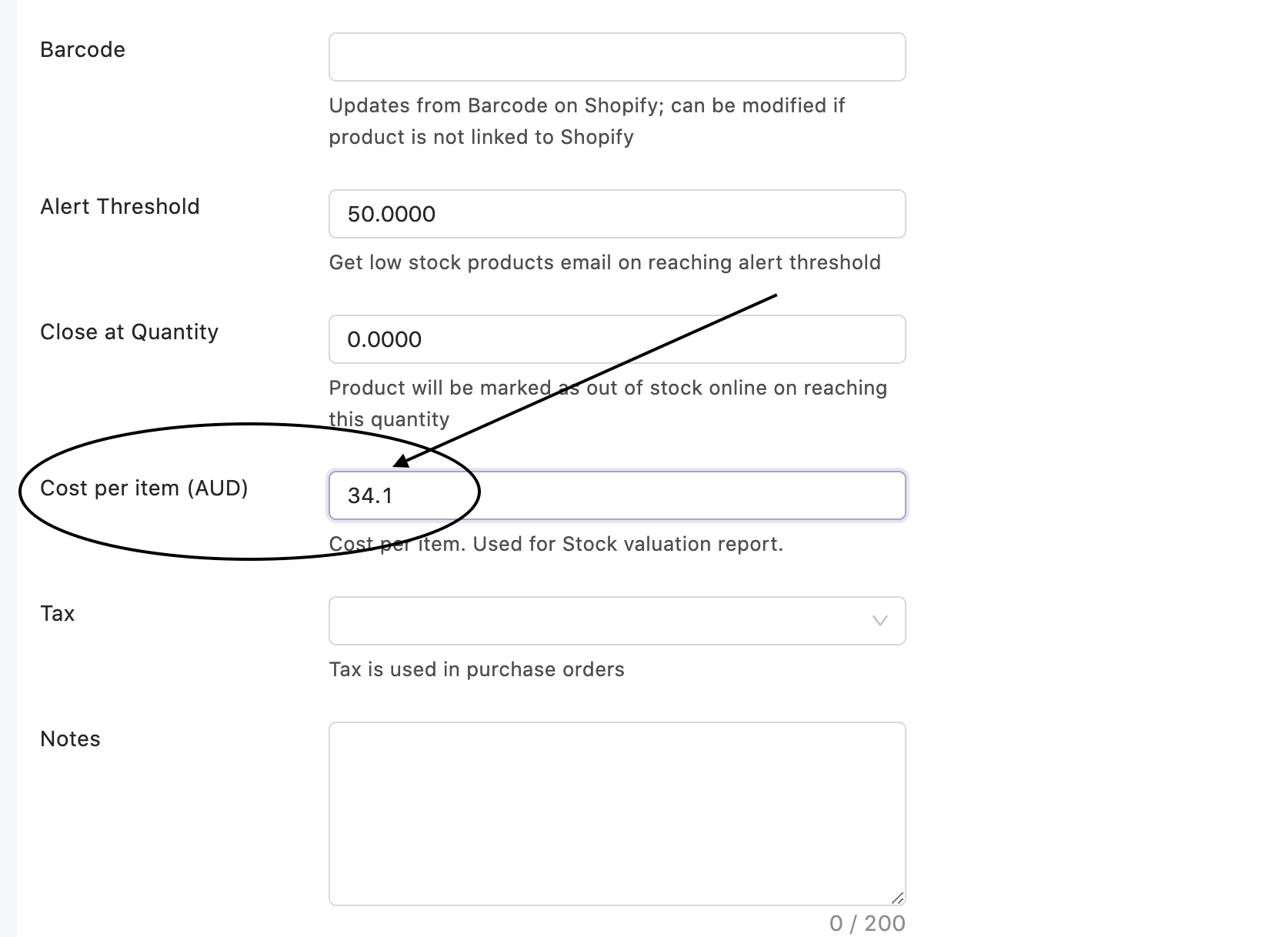## How are cost prices updated through Purchase Orders

We use the weighted average pricing model based on your existing inventory only.

When you receive items in a Purchase order, the cost of the product is updated as per the following calculation.

We calculate the per unit landed cost of the product in the PO.

`Per unit landed cost in the PO = ((line total - line tax)/line quantity + Extra shipping fee + Duties and charges are distributed over all products in PO based on line total) x currency conversion rate`

The currency conversion rate is 1 if the PO is made in default currency. If you are using any other currency, then you must update the correct conversion rate in PO.

After calculating the landed cost, we calculate the new cost price as follows

`New cost price = (existing inventory x existing cost price + quantity received in PO x per unit landed cost in PO)/(existing stock + quantity received)`

We do not update the cost of product if you have filled 0 in the purchase price of the product in PO

`Here's how the calculation for cost is done with a simple example -Lets says, existing inventory = 10 units existing cost = 10 USD/unit.Now you received 10 units @6 USD/unit.So the calculation which will happen isNew cost = (10 x 10 + 10 x 6)/(10 + 10) = 8 USD/unitLets say you sell some pieces and now you have 5 units left.You receive 10 units at 5 USD/unit.New cost = (5 x 8 + 10 x 5)/(5 + 10) = 6 USD/unitLets say you sell all pieces.Now you buy 10 units at 4 USD/unit.New cost = (0 x 6 + 10 x 4)/(0 + 10) = 4 USD/unit`